# 2023知到答案 化工原理（2）（全英文）（山东科技大学） 最新智慧树满分章节测试答案

## 绪论 单元测试

1、判断题：
Distillation is a kind of mass transfer operation. 精馏是一种质量传递操作。 （ ）

A:错
B:对

2、判断题：
Drying is not a kind of mass transfer operation. 干燥不是一种质量传递操作。（ ）

A:错
B:对

3、判断题：
The absorption process can be used in separating gas mixtures by their different solubility. 吸收可用于分离气体混合物。（ ）

A:错
B:对

## 第一章 单元测试

1、单选题：
The function of the condenser is to provide (B) product and ensure suitable liquid phase reflux. 冷凝器的作用是提供____产品及保证有适宜的液相回流。（ ）

C:Bottoms liquid phase塔底液相;
D:Bottoms vapor phase塔底气相;

2、单选题：
If the reflux ratio used in the continuous distillation operation is less than the original reflux ratio, then____. 操作中连续精馏塔，如采用的回流比小于原回流比，则____。（ ）

A:x D decreases and x W increases x D 减小，x W 增加;
B:Both x D and x W increase x D、x W 均增加;
C:X D and x W remain unchanged X D、 x W 均不变;
D:Unable to operate normally不能正常操作;

3、单选题：
In the calculation of continuous distillation of binary solutions, changes in the thermal state of the feed will cause changes in the following line ____. 二元溶液连续精馏计算中，进料热状况的变化将引起以下线的变化。（ ）

A:Equibrium line and Striping section operation line, 平衡线与提馏段操作线;
B:Equilibrium line and q line, 平衡线与 q 线;
C:q line and rectifying section operation line, q 线与精馏段操作线;
D:Striping section operation line and q line, 提馏段操作线与 q 线;

4、单选题：
Introducing reflux in distillation results in mass transfer between the falling liquid phase and the rising vapor phase, which increases the concentration of volatile components in the rising vapor phase. The most appropriate statement is ____. 精馏中引入回流，下降的液相与上升的汽相发生传质使上升的汽相易挥发组分浓度提高，最恰当的说法是____。（ ）

A:The entry of volatile components in the liquid phase into the vapor phase and the entry of non-volatile components in the vapor phase into the liquid phase must occur simultaneously. 液相中易挥发组分进入汽相和汽相中难挥发组分进入液相必定同时发生。
B:The difficult volatile components in the vapor phase enter the liquid phase汽相中难挥发组分进入液相;
C:The volatile and non-volatile components in the liquid phase enter the vapor phase simultaneously, but there are more volatile components among them液相中易挥发组分和难挥发组分同时进入汽相，但其中易挥发组分较多;
D:The volatile components in the liquid phase enter the vapor phase液相中易挥发组分进入汽相;

5、单选题：
Given the equation of the distillation section operating line as y=0.75x+0.24, the operating reflux ratio of the tower is ____. 已知精馏段操作线方程为： y=0.75x+0.24 ，则该塔的操作回流比为____。（ ）

A:4
B:0.98；
C:0.96
D:0.9 B .3

6、单选题：
Given the equation of the distillation section operating line as y=0.75x+0.24, then the top product concentration X D is ____. 已知精馏段操作线方程为： y=0.75x+0.24 ，塔顶产品浓度 X D 为____。（ ）

A:0.9 B .3
B:4
C:0.96
D:0.98；

## 第二章 单元测试

1、单选题：
The absorption rate is mainly determined by the diffusion rate through the double film, to improve the relative motion of the two fluids of gas and liquid, to improve the absorption effect, then to do____. 吸收速率主要决定于通过双膜的扩散速度，要提高气液两流体的相对运动，提高吸收效果，则要：（ ）

A:reduce the thickness of both gas and liquid film，减少气膜和液膜厚度
B:increase the thickness of the gas film and reduce the thickness of the liquid film，增加气膜厚度和减少液膜厚度
C:increase the thickness of gas and liquid film，增加气膜和液膜厚度

2、多选题：
If we adopt a gas adsorption process operating at a very high liquid flow rate, the consequences would be____. 对于气体吸收工艺，如果我们在很高的液体流速下运行，其后果是____。（ ）

A:low driving force, 驱动力低，
B:high operation cost, 运行成本高，
C:low equipment capital cost, 设备资本成本低，
D:low steam consumption蒸汽消耗量低

low equipment capital cost, 设备资本成本低，

3、单选题：
The packing characteristics that have a greater impact on the absorption operation are____．对吸收操作影响较大的填料特性是____。（ ）

A:the resistance to gas to be small
B:specific surface area and free volume;
C:mechanical strength;

4、判断题：
The greater the height of the total gas phase mass transfer unit HOG of the absorption tower, the more favorable to absorption. 吸收塔气相总传质单元高度HOG越大，对吸收越有利。（ ）

A:错
B:对

5、判断题：
Since the partial pressure of solute in the gas phase of the absorption process is always greater than the equilibrium partial pressure of solute in the liquid phase, the absorption operation line is always above the equilibrium line. 由于吸收过程气相中的溶质分压总大于液相中溶质的平衡分压，所以吸收操作线总是在平衡线的上方。（ ）

A:对
B:错

6、判断题：
For a certain operating conditions of the packed absorption tower, such as the tower material layer to increase some higher, the tower HOG will increase, NOG will remain unchanged. 对一定操作条件下的填料吸收塔，如将塔料层增高一些，则塔的HOG将增加，NOG将不变。（ ）

A:错
B:对

7、判断题：
Considering the total cost for gas absorption, as shown in the following figure, small liquid flow rate to increase the driving force of mass transfer from gas phase to liquid phase is always desired.考虑到气体吸收过程的总成本，如下图所示，总是希望用小的液体流速来增加从气相到液相的传质驱动力。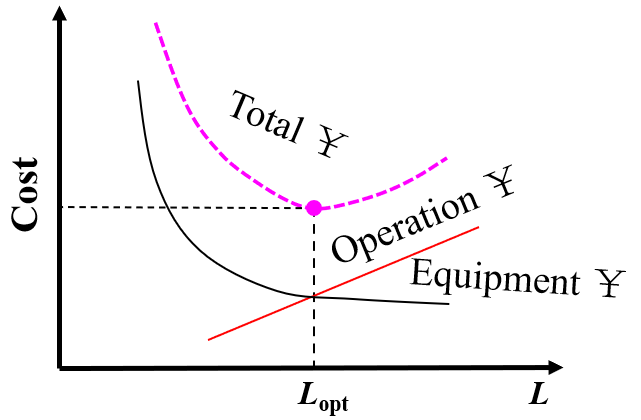（ ）

A:对
B:错

8、单选题：
We use ethylene diamine (EDA) to absorb SO2 in flue gas, as shown in the following figures. The flow rate of inert gas V'=1000kmol/h; the molar fraction of SO2 in the mixture gas before adsorption yb=0.04; the recovery φA=0.95; the molar fraction of SO2 in fresh liquid xa=0.005. The minimum ratio of solvent to inert gas (L'/V')min is 0.2kmol/h, and the practical ratio of solvent to inert gas L'/V'=1.3(L'/V')min. Find solvent flow rate L' and SO2 molar ratio after absorption Xb. 我们使用乙二胺（EDA）来吸收烟气中的SO2，如以下图所示。惰性气体的流量V'=1000kmol/h；吸收前混合气体中SO2的摩尔分数yb=0.04；回收率φA=0.95；新鲜液体中SO2的摩尔分数xa=0.005。溶剂与惰性气体的最小比例(L'/V')min取0.2kmol/h，溶剂与惰性气体的实际比例L'/V'=1.3(L'/V')min。求溶剂流量L'。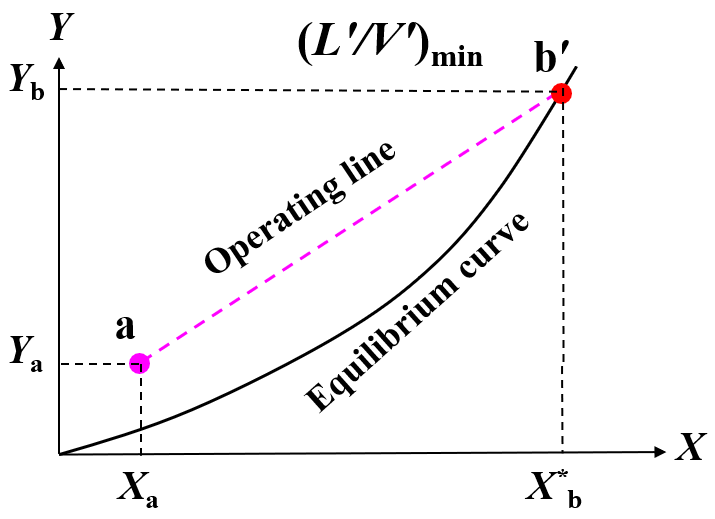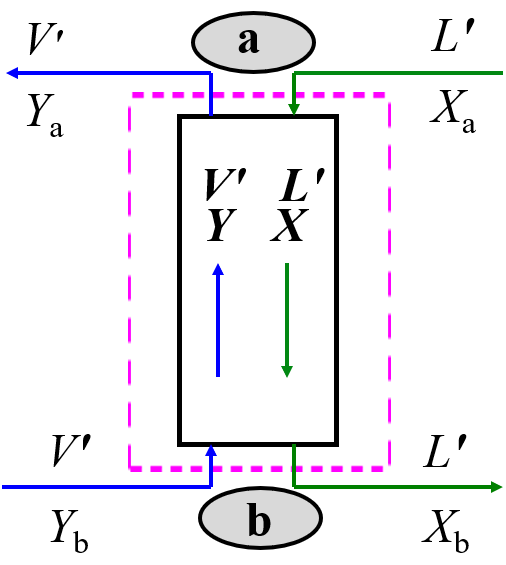（ ）

A:100kmol/h
B:430kmol/h
C:300kmol/h
D:230kmol/h

9、单选题：
We use ethylene diamine (EDA) to absorb SO2 in flue gas, as shown in the following figures. The flow rate of inert gas V'=1000kmol/h; the molar fraction of SO2 in the mixture gas before adsorption yb=0.04; the recovery φA=0.95; the molar fraction of SO2 in fresh liquid xa=0.005. The minimum ratio of solvent to inert gas (L'/V')min is 0.2kmol/h, and the practical ratio of solvent to inert gas L'/V'=1.3(L'/V')min. Find solvent flow rate L' and SO2 molar ratio after absorption Xb. 我们使用乙二胺（EDA）来吸收烟气中的SO2，如以下图所示。惰性气体的流量V'=1000kmol/h；吸收前混合气体中SO2的摩尔分数yb=0.04；回收率φA=0.95；新鲜液体中SO2的摩尔分数xa=0.005。溶剂与惰性气体的最小比例(L'/V')min取0.2kmol/h，溶剂与惰性气体的实际比例L'/V'=1.3(L'/V')min。求吸收后气体中SO2摩尔比Xb（ ）

A:0.862
B:0.345
C:0.632
D:0.137

10、判断题：
For a segment of packing tower for gas absorption, the integration of [1/(Y-Y*)]dY between Ya and Yb equals 3.8, where Y is the molar ratio of soluble component, Y* is the equilibrium molar ratio of the liquid, Ya and Yb are the molar ratios of solute in gas phase at the tower top and bottom respectively. Then the number of transfer units (NTU) with respect to gas phase overall driving force is 3.8. (True or false?) 对于用于气体吸收的填料塔，Ya和Yb之间的[1/(Y-Y*)]dY的积分等于3.8，其中Y是可溶组分的摩尔比，Y*是液体中的平衡摩尔比，Ya和Yb分别是塔顶和塔底气相中的溶质摩尔比。那么关于气相总传质驱动力的传质单元数（NTU）为3.8。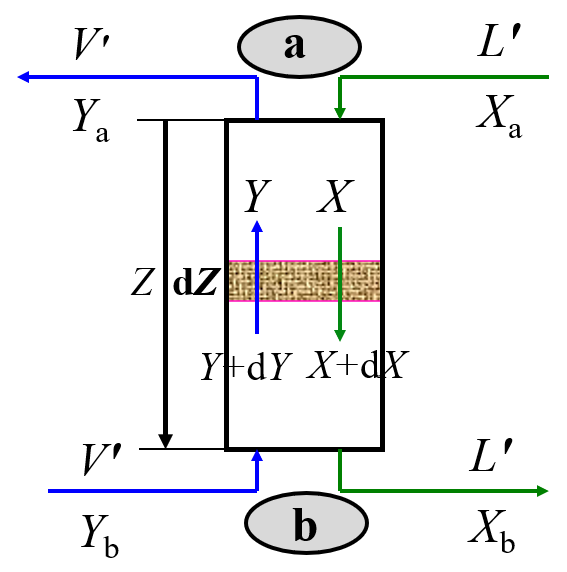（ ）

A:错
B:对

11、单选题：
For a plate type stripping tower, the number of theoretical plates (NTP) is determined by graphic method, as shown in the following figure. The molar ratios of solute in liquid and gas at the tower top and bottom Xa, Xb, Ya, Yb. The number of theoretical plates is: 对于板式汽提塔，理论塔板数（NTP）通过图形方法确定，如下图所示。塔顶和塔底的液体和气体中溶质的摩尔比为Xa、Xb、Ya、Yb。理论塔板数为：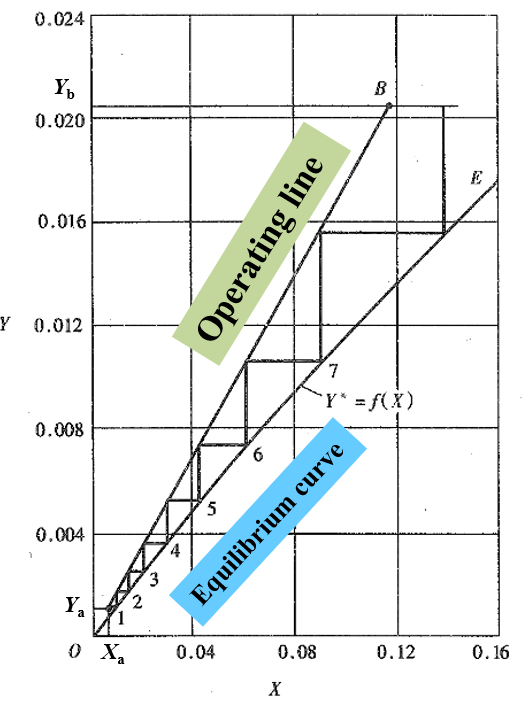（ ）

A:8
B:1
C:7.6
D:7